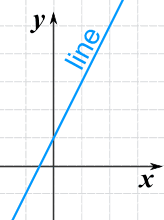# Introduction to Linear Equations

## What does it mean?

### Definitions:

From Wolfram MathWorld:

A linear equation is an algebraic equation of the form $$y = mx + b$$ involving only a constant and a first-order (linear) term, where m is the slope and b is the y-intercept.

Occasionally, the above is called a "linear equation of two variables," where y and x are the variables.

Linear -- from Wolfram MathWorld

Horizontal Line: $$y = 3 \space ( or \space\space any\space\space number )$$ Lines that are horizontal have a slope of zero. They have "run", but no "rise". The rise/run formula for slope always yields zero since rise = 0.

Vertical Line: $$x = -2 \space ( or \space\space any\space\space number )$$ Lines that are vertical have no slope (it does not exist). They have "rise", but no "run". The rise/run formula for slope always has a zero denominator and is undefined.

## What does it look like?

$$y = 3x – 6$$ $$y - 2 = 3(x + 1)$$ $$y + 2x - 2 = 0$$ $$5x = 6$$ $$\frac{y}{2} = 3$$$$y = 2x+1$$## You'll use it...

An equation of a function, C, which converts degrees Fahrenheit into degrees Celsius is an example of a linear function. Currency Exchange Rates. Cell Phone’s rate plans.

## Video

Watch a Khan Academy Video »
Length: 2:24

## Video

### Bridging Algebra and Geometry

Watch a Khan Academy Video »
Length: 11:22

## Video

### Graphing lines 1

Watch a Khan Academy Video »
Length: 9:50

## Video

### Plotting (x,y) relationships

Watch a Khan Academy Video »
Length: 4:29

## Video

### Graphing using X and Y intercepts

Watch a Khan Academy Video »
Length: 3:58

## Video

### Graphing a line in slope intercept form

Watch a Khan Academy Video »
Length: 3:02